##### Question

In: Statistics and Probability

# Suppose a random sample of n = 16 observations is selected from a population that is...

Suppose a random sample of n = 16 observations is selected from a population that is normally distributed with mean equal to 105 and standard deviation equal to 11. (use SALT)

(a) Give the mean and the standard deviation of the sampling distribution of the sample mean

x.

 mean standard deviation

(b) Find the probability that x exceeds 109. (Round your answer to four decimal places.)

(c) Find the probability that the sample mean deviates from the population mean μ = 105 by no more than 5. (Round your answer to four decimal places.)

## Solutions

##### Expert Solution

Solution :

Given that ,

mean =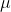= 105

standard deviation =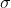= 11

n = 16

a)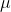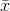== 105 and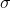=/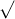n = 16 /16 = 4

b)

P(> 109) = 1 - P(< 109)

= 1 - P((-) /< (109 - 105) / 5)

= 1 - P(z < 1)

= 1 - 0.8413 Using standard normal table.

= 0.1587

Probability = 0.1587

c)

P(100 << 110) = P((100 - 105) / 4<(-) /< (110 - 105) / 4))

= P(-1.25 < Z < 1.25)

= P(Z < 1.25) - P(Z < -1.25) Using standard normal table,

= 0.8944 - 0.1056

= 0.7888

Probability = 0.7888

## Related Solutions

##### Suppose a random sample of n = 5 observations is selected from a population that is...
Suppose a random sample of n = 5 observations is selected from a population that is normally distributed, with mean equal to 7 and standard deviation equal to 0.33. (a) Give the mean and standard deviation of the sampling distribution of x. (Round your standard deviation to four decimal places.) mean standard deviation (b) Find the probability that x exceeds 7.3. (Round your answer to four decimal places.) (c) Find the probability that the sample mean x is less than...
##### Suppose a random sample of n = 5 observations is selected from a population that is...
Suppose a random sample of n = 5 observations is selected from a population that is normally distributed, with mean equal to 3 and standard deviation equal to 0.35. (a) Give the mean and standard deviation of the sampling distribution of x. (Round your standard deviation to four decimal places.) (b) Find the probability that x exceeds 3.3. (Round your answer to four decimal places.) (c) Find the probability that the sample mean x is less than 2.5. (Round your...
##### A random sample of n observations is selected from a population with standard deviation σ =...
A random sample of n observations is selected from a population with standard deviation σ = 1. Calculate the standard error of the mean (SE) for these values of n. (Round your answers to three decimal places.) (a) n = 1 SE = (b) n = 2 SE = (c) n = 4 SE = (d) n = 9 SE = (e) n = 16 SE = (f) n = 25 SE = (g) n = 100 SE =
##### A sample of n = 16 individuals is selected from a population with µ = 30....
A sample of n = 16 individuals is selected from a population with µ = 30. After a treatment is administered to the individuals, the sample mean is found to be M = 33. A. Assume that the sample variance is s2 = 16. Compute a single sample t-test to evaluate if the treatment produced a significant effect and calculate r2 to measure the size of the treatment effect. Use a two-tailed test with α = .05. B. Now, assume...
##### A sample of n = 16 individuals is selected from a population with µ = 30....
A sample of n = 16 individuals is selected from a population with µ = 30. After a treatment is administered to the individuals, the sample mean is found to be M = 33. A. Assume that the sample variance is s2 = 16. Compute a single sample t-test to evaluate if the treatment produced a significant effect and calculate r2 to measure the size of the treatment effect. Use a two-tailed test with α = .05. B. Now, assume...
##### A random sample of n = 25 observations is taken from a N(µ, σ ) population....
A random sample of n = 25 observations is taken from a N(µ, σ ) population. A 95% confidence interval for µ was calculated to be (42.16, 57.84). The researcher feels that this interval is too wide. You want to reduce the interval to a width at most 12 units. a) For a confidence level of 95%, calculate the smallest sample size needed. b) For a sample size fixed at n = 25, calculate the largest confidence level 100(1 −...
##### 1. A )When a sample of n observations are selected from population that has a mean...
1. A )When a sample of n observations are selected from population that has a mean equal to μ, then the sampling distribution of sample means will have a mean... that may be larger or less than μ , depending on the size of the sample, n greater than μ less than μ equal to μ b) When a sample of n observations are selected from population that has a mean equal to μ and a standard deviation equal to...
##### A random sample of size n1 = 16 is selected from a normal population with a...
A random sample of size n1 = 16 is selected from a normal population with a mean of 74 and a standard deviation of 9. A second random sample of size n2 = 7 is taken from another normal population with mean 68 and standard deviation 11. Let X1and X2 be the two sample means. Find: (a) The probability that X1-X2 exceeds 4. (b) The probability that 4.8 ≤X1-X2≤ 5.6. Round your answers to two decimal places (e.g. 98.76).
##### A random sample of 120 observations is selected from a binomial population with an unknown probability...
A random sample of 120 observations is selected from a binomial population with an unknown probability of success ?. The computed value of ?̂ is 0.7. (1)    Test ?0:?=0.55 against ??:?>0.55. Use ?=0.01. test statistic ?= critical ? score      (2)    Test ?0:?=0.5 against ??:?<0.5. Use ?=0.05. test statistic ?= critical ? score      (3)    Test ?0:?=0.55 against ??:?≠0.55. Use ?=0.01. test statistic ?= positive critical ? score     negative critical ? score
##### A random sample of nequals100 observations is selected from a population with muequals31 and sigma equals...
A random sample of nequals100 observations is selected from a population with muequals31 and sigma equals 24. Complete parts a through f. Find ​P(x overbarxgreater than or equals≥​28).